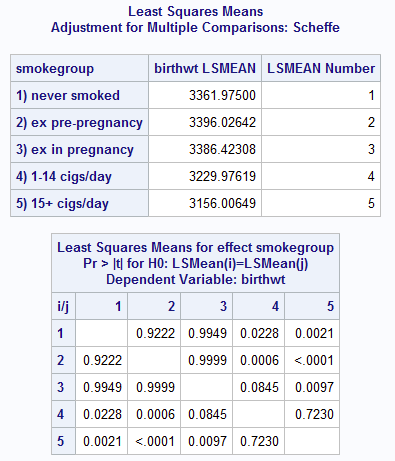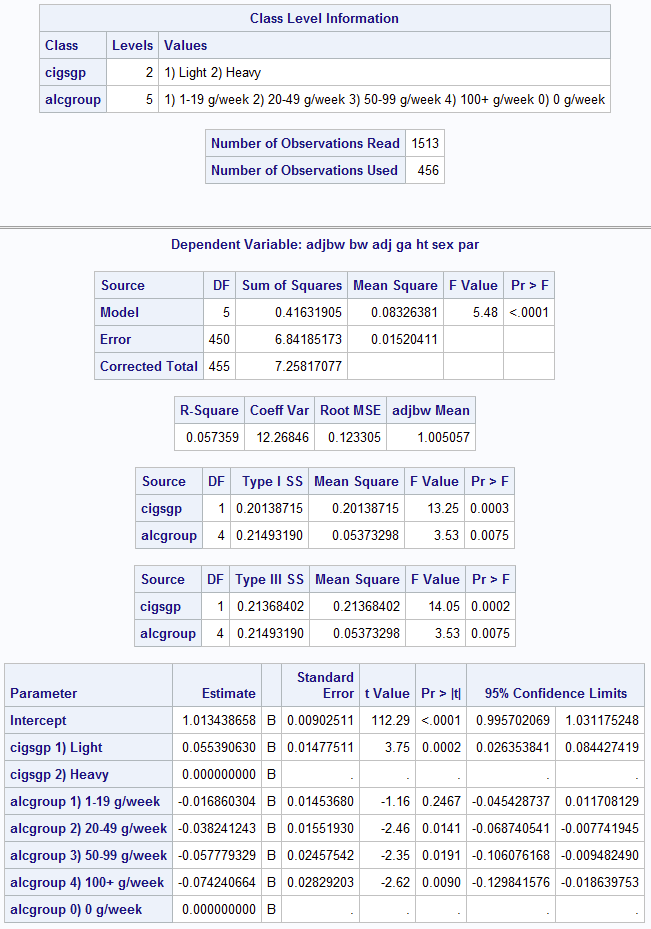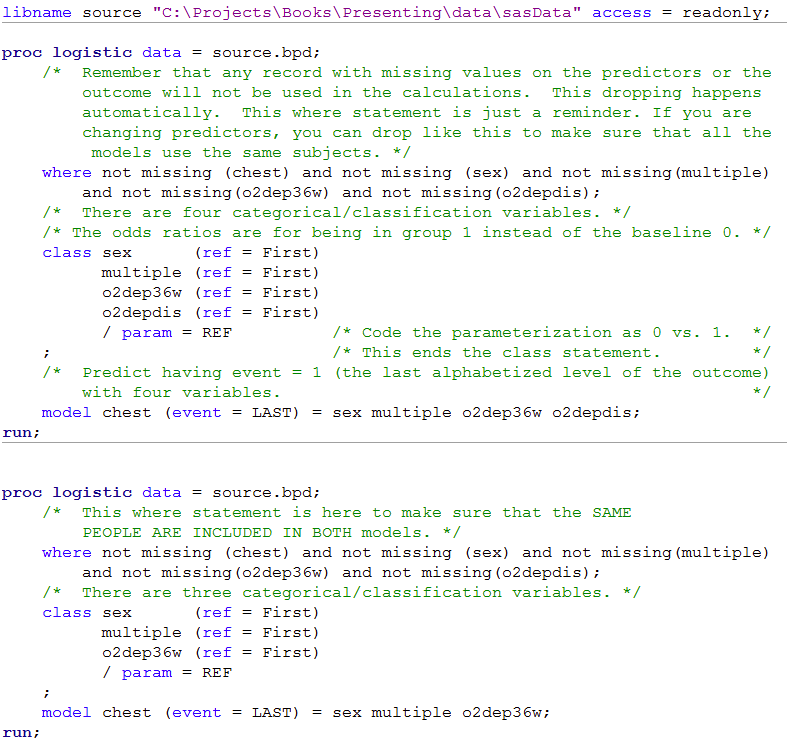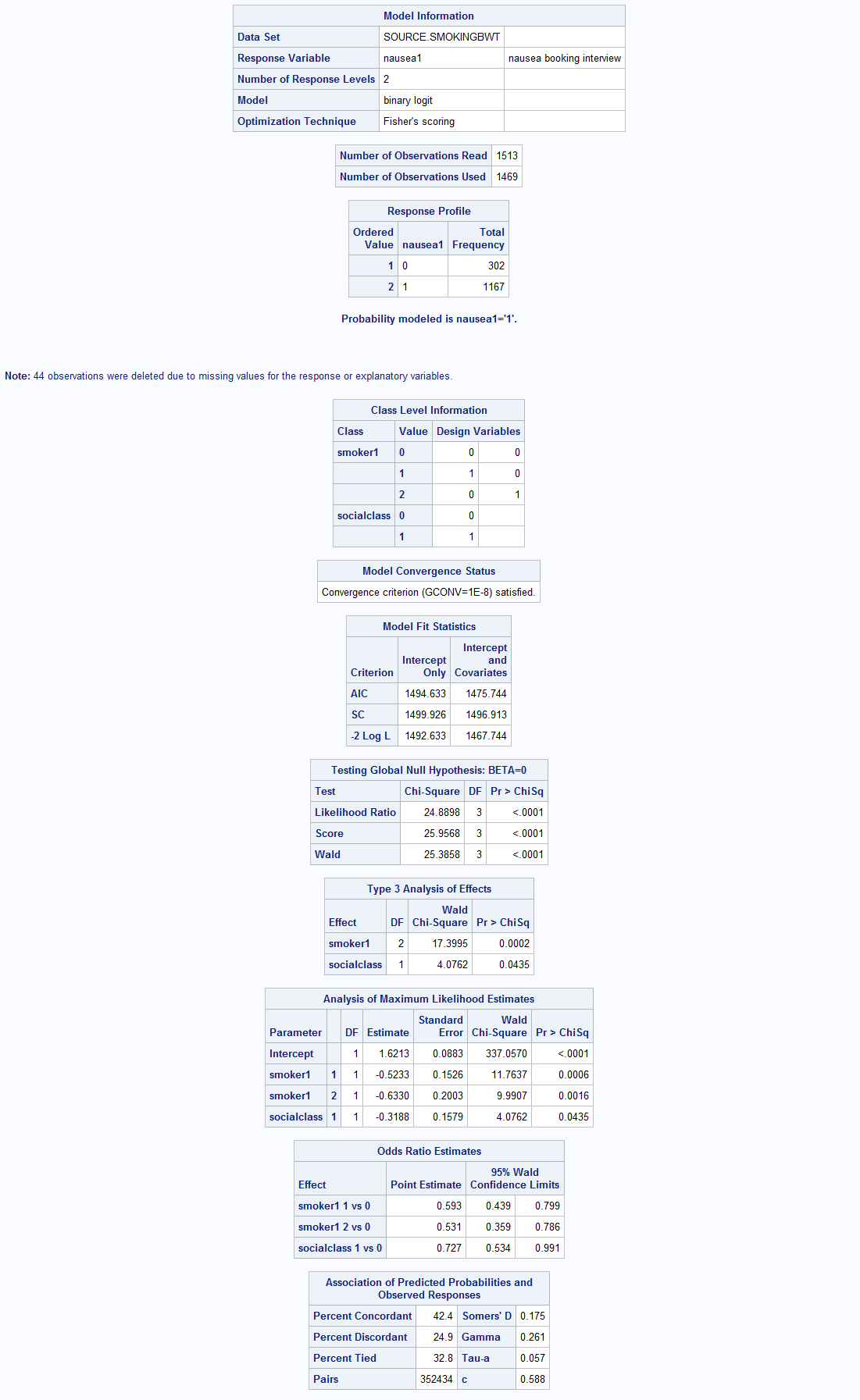﻿ Chapter 10: Multifactorial analyses using SAS

# Chapter 10: Multifactorial analyses using SAS

### Box 10.3 Presenting the results for one-way analysis of variance### Figure 10.1 and Box 10.3 Code### Box 10.5 Presenting the results of multiple regression### Figure 10.2 and Box 10.5 Code### Box 10.8 Presentation of multiple regression with categorical predictor variable### Box 10.8 Figure 10.3 Code### Box 10.12 Presenting the results for logistic regression### Figure 10.5 Code### Box 10.14 Presenting logistic regression with categorical predictor variables### Figure 10.6 and Box 10.14 Code